Trial ends in

# Easy Measurement of Diffusion Coefficients of EGFP-tagged Plasma Membrane Proteins Using k-Space Image Correlation Spectroscopy

Published: May 10, 2014 doi: 10.3791/51074

### Summary

This paper provides a step by step guide to the fluctuation analysis technique k-Space Image Correlation Spectroscopy (kICS) for measuring diffusion coefficients of fluorescently labeled plasma membrane proteins in live mammalian cells.

### Abstract

Lateral diffusion and compartmentalization of plasma membrane proteins are tightly regulated in cells and thus, studying these processes will reveal new insights to plasma membrane protein function and regulation. Recently, k-Space Image Correlation Spectroscopy (kICS)1 was developed to enable routine measurements of diffusion coefficients directly from images of fluorescently tagged plasma membrane proteins, that avoided systematic biases introduced by probe photophysics. Although the theoretical basis for the analysis is complex, the method can be implemented by nonexperts using a freely available code to measure diffusion coefficients of proteins. kICS calculates a time correlation function from a fluorescence microscopy image stack after Fourier transformation of each image to reciprocal (k-) space. Subsequently, circular averaging, natural logarithm transform and linear fits to the correlation function yields the diffusion coefficient. This paper provides a step-by-step guide to the image analysis and measurement of diffusion coefficients via kICS.

First, a high frame rate image sequence of a fluorescently labeled plasma membrane protein is acquired using a fluorescence microscope. Then, a region of interest (ROI) avoiding intracellular organelles, moving vesicles or protruding membrane regions is selected. The ROI stack is imported into a freely available code and several defined parameters (see Method section) are set for kICS analysis. The program then generates a "slope of slopes" plot from the k-space time correlation functions, and the diffusion coefficient is calculated from the slope of the plot. Below is a step-by-step kICS procedure to measure the diffusion coefficient of a membrane protein using the renal water channel aquaporin-3 tagged with EGFP as a canonical example.

### Introduction

The four dimensional spatiotemporal organization and lateral mobility of plasma membrane proteins are tightly regulated and may play a role in protein function, activity and protein-protein interactions. Lateral diffusion of plasma membrane proteins, has traditionally been studied by calculating diffusion coefficients for single particles from time-lapse imaging of quantum dot or dye labeled plasma membrane proteins2-4. This approach requires insertion of an extracellular tag in the plasma membrane protein for quantum dot or dye labeling, which can compromise protein folding and function and thus, cannot be achieved for some proteins. The steric volume of a quantum dot has been shown to slow down diffusion of the protein5 and moreover, only a tiny fraction of the proteins in the population are labeled with quantum dots, and it is not known if this fraction is representative for the total pool of plasma membrane proteins. Single particle tracking (SPT) measurements of diffusion coefficients from image series of quantum dot labeled proteins involves mapping the imaged peak positions of the particles with two dimensional Gaussian fits followed by extensive analysis algorithms. The analysis is computationally very intensive, requiring extensive non-linear curve fitting in each image frame of a time-lapse sequence to approximations of the microscope point spread function (typically two dimensional spatial Gaussians) and subsequent linking of particle positions into particle trajectories describing the motion of single molecules6,7.

A recently developed image correlation technique, k-Space Image Correlation Spectroscopy (kICS) enables relative simple measurements of diffusion coefficients of fluorescently tagged plasma membrane proteins. The possibility to routinely calculate diffusion coefficients of membrane proteins labeled with fluorescent proteins by kICS is a unique tool which holds several advantages over traditional quantum dot SPT analysis: No insertion of extracellular tags and time consuming extracellular labeling is required (cell lines expressing fluorescent proteins may be used); diffusion coefficients are extracted from the total pool of fluorescent proteins compared to a subset labeled with quantum dots; analysis is simple without the need to track single proteins and the analysis can be performed using an existing code with no requirement for additional user programming. The method is rapid because it is an averaging technique which enables fast computation and calculation of diffusion coefficients. These rapid diffusion measurements for the protein population complement the more detailed molecular transport information obtained for a subset of the population by the painstaking SPT methods.

kICS time correlates fluorescence microscopy image sequences that have first been transformed to Fourier space, and thereby separates fluorescence fluctuations due to photophysics from those due to molecular transport1. As a result, kICS can determine the number density, flow speed, and diffusion of fluorescently labeled molecules while being unbiased by complex photobleaching or blinking of the fluorophores. This makes kICS a useful tool for quickly determining the diffusion dynamics of fluorescently-labeled cell membrane proteins without the need to custom write algorithms. Besides fluorescently labeled proteins, kICS can also be applied to quantum dot-labeled membrane proteins8.

This paper provides a step-by-step introduction of how to use kICS to extract diffusion coefficients of EGFP-tagged plasma membrane proteins by demonstrating how to place the crop, how to use the code and how to evaluate the generated plots, from which diffusion coefficients are extracted. As an example, data obtained by spinning disk-microscopy set in semi-total internal reflection fluorescence (TIRF) mode, of a renal water channel protein aquaporin-3 (AQP3) tagged with EGFP is presented.

### Protocol

Acquisition of EGFP-tagged proteins in the plasma membrane for kICS analysis can be done on an epifluorescence microscope, a TIRF setup or on a spinning disk microscope set to acquire images of the membrane of interest. The camera pixel size as well as the time between image frames is needed for the analysis. Image sequences of 100-1,200 frames at a frame rate of 4-30 Hz can be used for the analysis. During the acquisition, it is important to keep the membrane in focus throughout the duration of the imaging and focus on a fraction of the flat membrane in which the fluorescence is uniformly distributed. Moving vesicles, protruding membrane regions and cell organelles can be cropped out later in the analysis process. The acquisition time should be chosen so that there is no drift or movement of the cell.

To get the kICS code scripts for MATLAB, contact Paul Wiseman (Paul.Wiseman@McGill.ca). The first time the kICS code is used, open MATLAB and click file, then set path, then click add with subfolders and find the folder on the computer in which the kICS code is located. Then click ok, save and close. Now continue with the analysis of crops described in the next points.

1. Import the Image Sequence of Interest into an Imaging Analysis Program as a TIFF Stack

Save the file with the name: stack1.tif.

1. Select a rectangular region of interest (ROI) at the flat part of the membrane excluding moving cell organelles and protruding membrane regions. The crop size is chosen so that enough statistics are generated for good k2 plots.
2. Crop the region and save the crop as a TIFF file in an appropriate folder. If there are several crops from the same movie, use a number increment, e.g. “stack1crop.tif”, “stack1crop2.tif”, ” stack1crop3.tif”.
3. Place the folder containing the crops locally on the computer, not on a server, while doing kICS analysis.

2. Import the Crops into the Analysis Software One by One to Perform the Analysis

1. Open MATLAB and type ”icsgui” in the command window and press ENTER. ICS GUI is the executable name for the image correlation spectroscopy graphical user interface program.
2. In the ICS GUI click the “kICS” tab. A screen shot of the analysis window is shown in Figure 1.
3. Find the folder with the folder name “MATLAB code” and scroll to the file with the file name “Scripts” and open this by double clicking it. Alternatively, right click the file and select “open with MATLAB” or go to file, open in MATLAB and open the scripts file. This file is called Editor.
4. In the Editor scroll to “Load kICS data sets”, then copy or type file name and folder location into the command line which begins with img = e.g. “C:\Users\Documents\AQP3dynamics\stack1crop.tif”. In the command line below the folder location set the number of frames to be analyzed – e.g. [1:500]. Use the same settings for all consecutive movies in the data series. If there is focus drift in later frames, these should be excluded from the analysis.
5. In the editor click “save” and then click “evaluate cell”. The dataset is now loaded into into the analysis software.
6. In the kICS Analysis window in the GUI, enter the settings for the analysis:
1. Unclick “T cell” boxes.
2. Enter the number of time lags to be analyzed. The number of time lags (τ) will have effect on the diffusion plot and must be chosen so that the diffusion plot is linear. Start with entering 25 and then decrease to where the diffusion plot is linear. τ is the number of time-lags the kICS analysis compares and a time lag is the time between one frame and the next. The smallest value which gives a linear plot should be selected. The maximum number of time lags is chosen so that a linear fit is obtained, choosing a higher value will produce data which cannot be line fitted.
3. Enter the maximum k2 value (see circle 1 in Figure 1), it is usually 20-50. A good k2 plot is a plot in which there are many data points which are distributed along a line. Start with entering 70 and then decrease after having evaluated the k2 and diffusion plots and select the lowest value where the data points cluster around a straight line. The k2 value is the squared magnitude of the spatial k-vector in Fourier space.
Initially, the analysis should be repeated until the best values are determined, then use the same settings for all consecutive movies in the data series but always check that the selected settings give a good fit to the data: good k2 plots and a linear diffusion plot.
4. Click “Store settings and proceed”.
5. Click “yes” to show all graphs.
6. Click “Load image series” (see circle 2 in Figure 1), then click “workspace”.
7. Select “imgSerCrop”, then click “Import from Workspace”.
8. Enter the imaging system collection settings. In the box “pixel size” enter the projected pixel size for the imaging system at which the image stacks are acquired. For AQP3-EGFP, 0.111 was used. In the box “Enter the time (s) between frames”, 0.1150 was used for AQP3-EGFP.
9. Click “select ROIs”, enter “1”, click ok and “use whole image”.
10. Click “Do kICS analysis” and click yes to do “immobile filtering”. Results will open automatically as images.
11. Minimize the settings window, minimize the cell info window, and minimize the photophysics window. In the dynamics window, check that the dynamics plot shows a linear fit of the data to a line with a negative slope meaning that the data points correlate and free diffusion can be assumed. Record the diffusion coefficient for the specific time lag and k2 settings (it is not necessary to note the standard deviation of the fit). The data points in the k2 plots must be clustered around the line for the given time lags.
12. Tick box “Save open figures as images”, then click “Save open figures as images” to save result files. Save in a new folder under C:\Users\Documents\AQP3diffusion. Click “clear current data” and continue with analysis of the next crop.

3. Average of the Calculated Diffusion Coefficient is Calculated to Get an Overview of the Analyzed Data

1. Enter the individual diffusion coefficients from each ROI in a spreadsheet (make sure to note the τ and k2 values), use the names of the crops as set above.
2. Calculate the average diffusion coefficient and standard deviation using a spreadsheet program.
3. Perform a Kolmogorov-Smirnov test or students t-test to evaluate statistical differences using a 5% significance level. Quantitave comparisons between cells (for example treated vs. nontreated) can be made.
4. Open the spreadsheet version of the diffusion plots which was generated by the analysis software for each crop, and average the data for each condition for each time lag. Generate a new averaged diffusion plot for presenting in papers or presentations.

### Representative Results

To acquire suitable image stacks to be analyzed with kICS, different microscope systems can be used. It is possible to analyze image sequences from an epi-fluorescence microscope as well as a TIRF or spinning disk setup. The membrane must be flat without any large moving cell organelles or moving vesicles/objects. This paper presents a time lapse image sequence of AQP3 tagged with EGFP in live MDCK cells imaged on a spinning disk microscope with focus set to the plasma membrane at a frame rate of 9.2 Hz. The focus was set at the basal (bottom) plasma membrane. The data has recently been published in AJP Cell13.

Figure 2A shows an image of the cell. The scalebar is 10 mm and example ROI crops are highlighted in rectangles. For the selection of crops it is important to crop at the periphery of the cell where the membrane is uniform and flat so only a two-dimensional diffusion is visualized. Cell organelles, vesicles and membrane projections should be avoided and it is important for the analysis that there is no drift during the time lapse. Examples of crops which would be excluded from analysis are presented in Figures 2B and 2C. Moving vesicles contribute to the correlation (Figure 2B) and holes in the membrane which in this case move slightly (Figure 2C) obviously should not be included in the analysis. It is also important that the ROI crop is large enough to have sufficient spatial sampling for the analysis (for imaging with a high NA objective lens a minimum linear size of 32 pixels is a reasonable guideline). For this dataset a 60X (NA 1.40) was used.

Figure 3A shows the diffusion plot of one crop generated with kICS. This method can be used to find the diffusion coefficient of a protein tagged with EGFP (or another fluorescent protein), a dye or quantum dots. The slope of the curve in the diffusion plot is simply the negative of the diffusion coefficient. In this case, the diffusion coefficient of AQP3-GFP was 0.0104 ± 0.0040 µm2/s. Figure 3B is a calculated diffusion plot with too many time lags, in this case the analysis was redone but with fewer time lags so that the diffusion plot is linear.

Figure 4A presents a typical k2 plot. This is the radially averaged and ln transformed correlation curve for a selected time lag. From these k2 plots the slope is plotted versus time lags and the result is the diffusion plot as shown in Figure 3A. When inspecting the k2 plots, it is important that the fit is evaluated. Figure 4B is an example of a k2 plot for which it can be concluded that the crop is too small and could not be analyzed as it should have a negative slope and decay linearly to a noise floor. Figure 4C is an example of a k2 plot which needs to be reanalyzed with a reduced maximum k2 value as the fit is no longer good (as judged by the increasing residuals at larger k2 values). In this case the analysis must be run again entering a lower maximum k2 value, in this case 25.

Figure 5 shows an averaged diffusion plot averaged over 10 crops. The diffusion plot was found in the excel file from the analysis, and all the diffusion plots can now be averaged for all crops from the same experiment. In the resulting diffusion plot different treatments of the cell can be combined in the same figure. Parallel trend lines are equal diffusion coefficients and non parallel trend lines indicate unequal diffusion. In this figure, the AQP3-EGFP diffusion coefficient is calculated (data from AJP Cell13).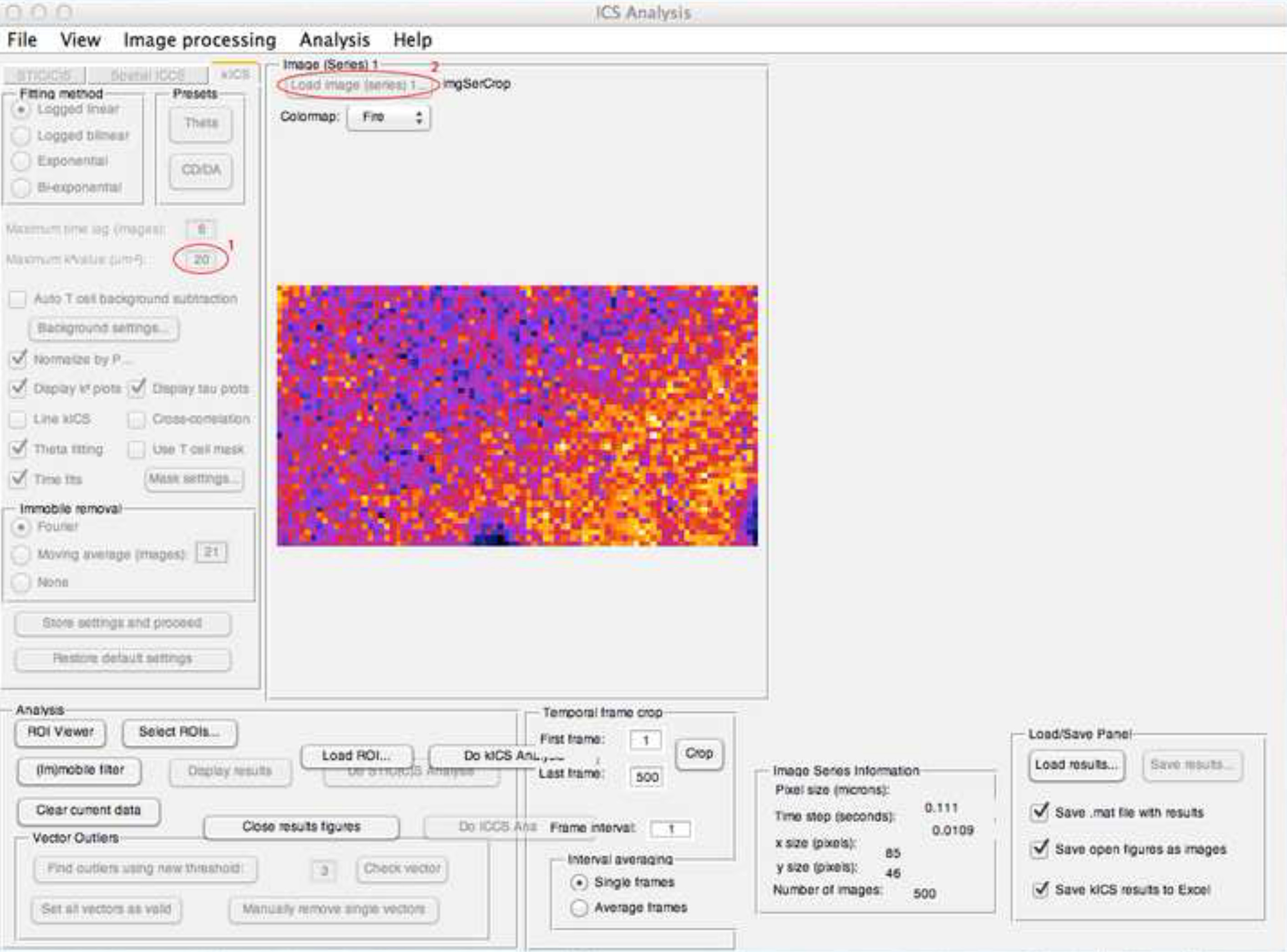Figure 1. Screen shot of the kICS analysis window. In this window the kiCS analysis is done. The circles indicate which buttons to press for the individual steps in the protocol. Click here to view larger image.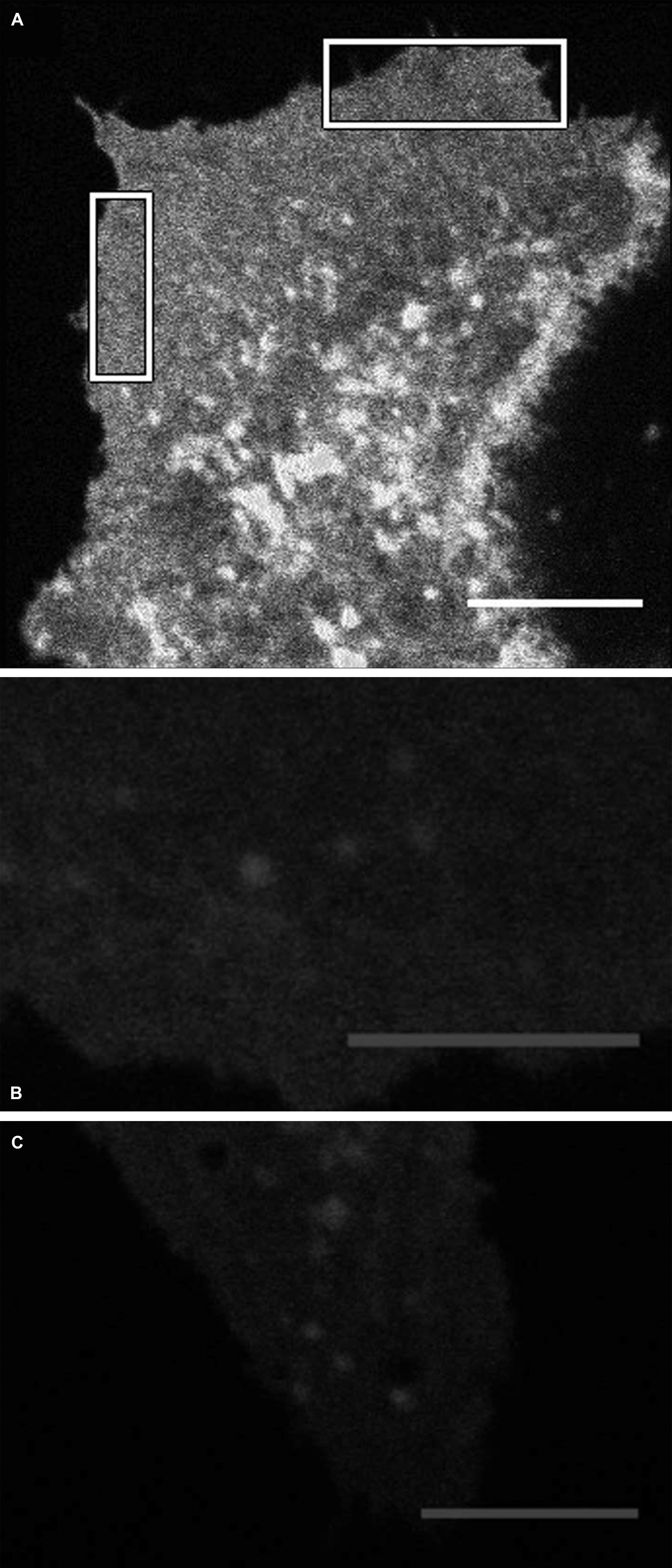Figure 2. Spinning disk image of a cell expressing GFP. (A) Representative image of an MDCK cell stably expressing AQP3-GFP and representative crops shown. The image is acquired on a spinning disk microscope with focus close to the basal plasma membrane. (B) Representative crop, which was excluded from analysis in kICS since there are moving vesicles, which can contribute to the diffusion coefficient. (C) An example of a crop in which there is a hole in the membrane, thus, this crop was excluded from analysis. All scale bars are 10 μm. Click here to view larger image.Figure 3. Diffusion plots. (A) A representative diffusion plot in which all point are close to the line fit. This is a good diffusion plot because the data are linear. (B) An example of a diffusion plot for which the analysis must be redone using a lower number of timelags. Click here to view larger image.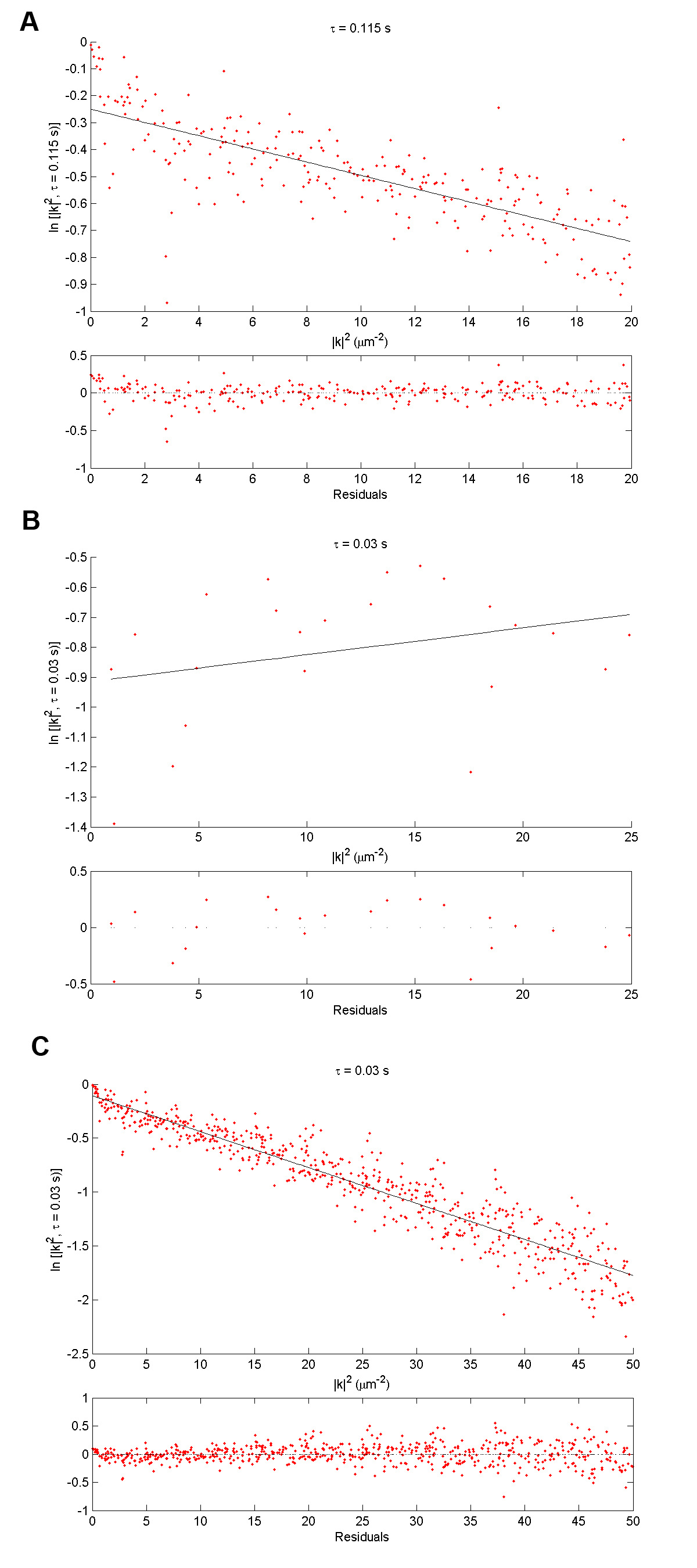Figure 4. Examples of k2 plots. (A) An example of a good k2 plot in which the data points are equally distributed on either side of the line fit. (B) An example of a k2 plot for a crop smaller than the optimal size. In this k2 plot, the slope of the line fit is positive, it should be negative and therefore the crop is excluded from the analysis.  (C) This k2 plot shows that  the analysis must be redone with a lower maximum k2 value in order to select the part of the k2 plot were the data are equally distributed on either side of the line fit. Click here to view larger image.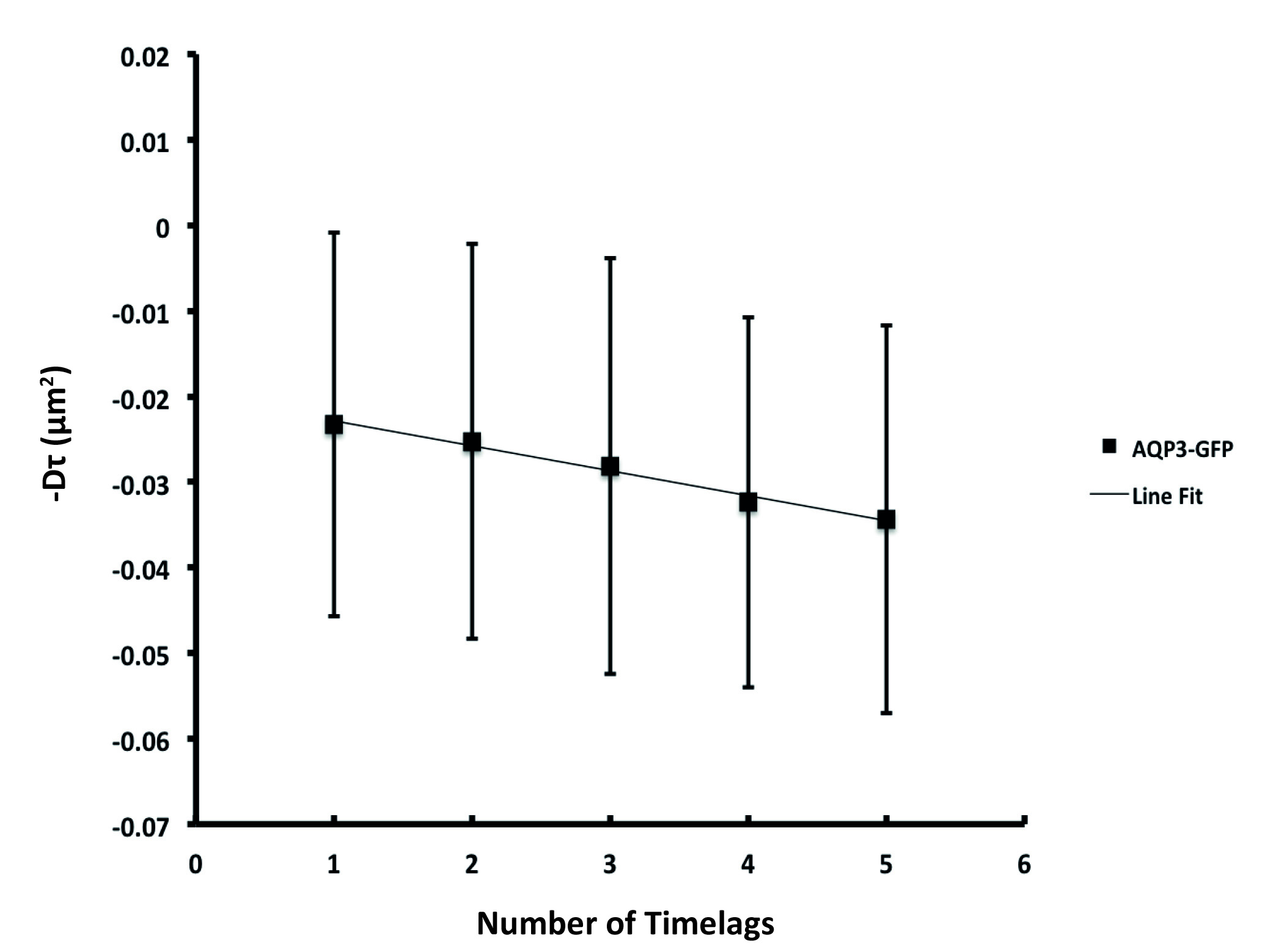Figure 5. Averaged diffusion plot. An averaged diffusion plot that was averaged over 10 crops.

### Discussion

This paper presented a detailed step-by-step overview of how to apply the kICS analysis method to determine diffusion coefficients from microscopy images of proteins tagged with fluorescent proteins. The analysis is independent of probe choice with a very broad dynamic range in labeling density and can thus also be applied to proteins labeled with quantum dots as well as dyes and fluorescent proteins such as EGFP.

To calculate valid diffusion coefficients for the proteins, there are several critical steps, which have been optimized. It is essential to image labeled proteins on the membrane only. If there are additional objects like moving vesicles or cell organelles in the image sequence to be analyzed, these will contribute to the calculated diffusion coefficient which results in under- or overestimates of the diffusion coefficient.

Following the steps listed above, the GUI code for kICS is user friendly and analysis is rapid. It includes several averaging steps: including both the circular averaging and the calculation of the slope of slopes diffusion plot as function of time lags, which facilitates quick generation of an average and statistically significant value for the diffusion coefficient. Thus, compared to the extensive analysis needed to calculate diffusion coefficients from SPT experiments, kICS is user friendly, rapid and can be performed without specialized training in biophysics.

If the analysis results in a negative diffusion coefficient or a horizontal diffusion plot, trouble-shooting includes visual inspection of the crop to see if it fulfills all requirements described here. Crops which are too small can yield unphysical negative diffusion coefficients and must be discarded. In order to calculate a diffusion coefficient, it is often possible to make a new crop elsewhere in the dataset which is suitable for analysis or simply increase the crop size if possible.

This technique can be applied to most datasets but currently, kICS analysis can only calculate an average diffusion coefficient and cannot distinguish different protein migration patterns or generate trajectories. Using kICS, diffusion coefficients can easily be extracted and diffusion of a protein can be compared for example between treated and non-treated cells or between a wild-type and a mutant protein, which will reveal if treatment of mutations induce differences in diffusion and hence potentially in protein-protein and/or protein-lipid interactions. Plasma membrane diffusion of AQP2 measured by FRAP was shown to decrease upon addition of forskolin, a cAMP agonist10, and moreover, in the ER, a mutated version of AQP2 causing nephrogenic diabetes insipidus, diffused differently than wildtype AQP2 9.

Although it is possible to calculate diffusion coefficients from single particle data using mean squared displacement, step size analysis or particle image correlation spectroscopy2,11,12, these analysis methods can be computationally demanding and often require custom written computer algorithms. The advantage of the kICS protocol presented here is that it is relatively independent of labeling density and computationally easy. This walk through of kICS analysis will thus be useful for many cell biologists, who can easily apply it to their membrane protein of interest.

### Disclosures

The authors declare that they have no competing financial interests.

### Acknowledgments

This work was supported by a Lundbeck Junior Group Leader Fellowship to LNN. PWW acknowledges grant funding support from the Natural Sciences and Engineering Research Council of Canada (NSERC). We also thank the Danish Molecular Bioimaging Center at University of Southern Denmark for access to spinning disk microscopy.

### Materials

 Name Company Catalog Number Comments DMEM Gibco 31600-083 FBS Invitrogen 10082147 Penicilin Sigma 13752 Kanamycin Gibco 15160070 Streptomycin Gibco 11860038 Phenol red free medium Gibco 11880-028 DMSO Sigma D8418 HEPES Gibco 15630056 Apparatus Spinning Disk Microscope Nikon Ti Eclipse EMCCD Camera Andor Ixon+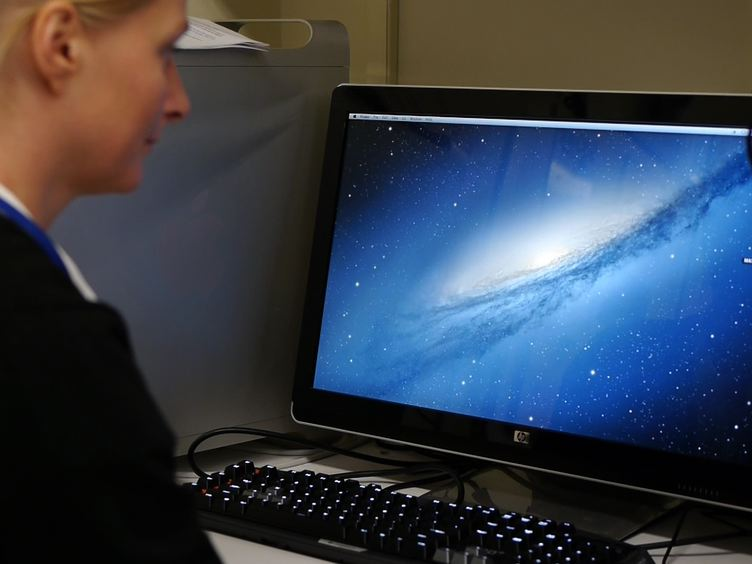Play Video
DOIX